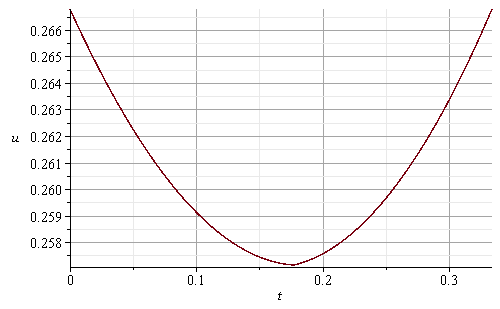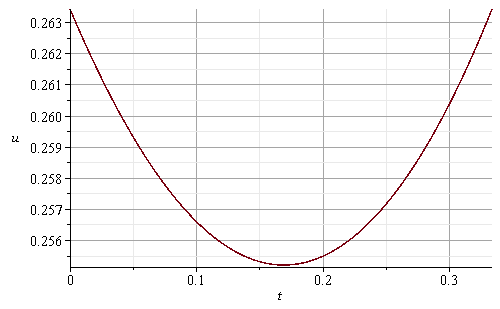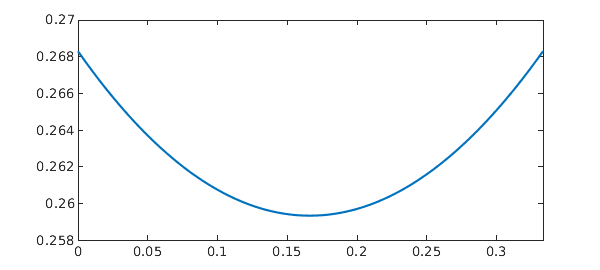## Exporting Graphs to PDF Using Maple Code?...

The following code effectively converts the image to the JPG format.
```with(GraphTheory):
s:=DrawGraph(CompleteGraph(5),size=[250,250])
Export("D:\\s1.jpg",s)
```

But I would like to export it using PDF format. However, the modified code below seems to be quite unsuccessful. I am aware that Maple has export options in the front end, but I still prefer to use code for this purpose.

`Export("D:\\s1.pdf",s)`

Error, (in Export) invalid input: member received _ImportExport:-InfoTable["PDF"], which is not valid for its 2nd argument, s

There are two reasons for this.

```with(GraphTheory):
Graphs:=[NonIsomorphicGraphs(6,8,output=graphs,outputform = graph)]:
num_g:=nops(Graphs):
num:=ceil((num_g)/5.):
Matrix (num,5,(i,j)->`if`((i-1)*5+j<=num_g, DrawGraph(Graphs[(i-1)*5+j],size=[250,250] ,overrideoptions ,showlabels=false,style=planar, stylesheet =  [
vertexcolor     = orange
,vertexfontcolor = black
,vertexborder    = false
,edgethickness   = 0.6
,edgecolor       = MidnightBlue
,vertexshape     =  "circle"
,vertexfont      = [Arial, 4],
vertexthickness=5], caption = cat(H__,5*(i-1)+j),captionfont=["ROMAN",7]),plot(x = 0 .. 1, axes = none))):
DocumentTools:-Tabulate (M1[1..5,.. ],widthmode=percentage ,width=80 , exterior =all):

```

## Is StringTools['StringBuffer'] outdated now?...

Since strings are not mutable objects in Maple, the package provides two procedures, `StringTools:-OldStringBuffer` and `StringTools:-StringBuffer`, which appear heavily correlated with Java's  and .

The help page of StringBuffer claims that use of a is much more efficient than the naive approach:

```(*
`G` and `F` are taken from the link above.
*)
G := proc()
description "extremely inefficient string concatenator";
local   r;
r := proc()
if nargs = 0 then
""
elif nargs = 1 then
args[ 1 ]
else
cat( args[ 1 ], procname( args[ 2 .. -1 ] ) )
end if
end proc;
r( args )
end proc:
# # This can be transformed into an O(1) algorithm by passing a string buffer to the recursive calls.
F := proc()
description "efficient version of G";
local    b, r;
b := StringTools:-StringBuffer();
r := proc()
if nargs = 1 then
b:-append( args[ 1 ] )
else
b:-append( args[ 1 ] );
procname( args[ 2 .. -1 ] )
end if
end proc;
r( args ):-value()
end proc:
s := 'StringTools:-Random(10, print)' \$ 1e4:
NULL;
time(G(s));
5.375

time(F(s));
1.125

```

But why not use the built-in `cat` directly?

```time(cat(s));
0.

time(StringTools:-Join([s], ""));
0.

```

Clearly, this is even more efficient

Here is the last example in that link.

```FilterFile := proc( fname::string, filter )
local   b, line;
b := StringTools:-StringBuffer();
do
if line = 0 then break end if;
b:-append( filter( line ) )
end do;
b:-value()
end proc: # verbatim
filename__0 := FileTools:-JoinPath(["example", "odyssey.txt"], 'base' = 'datadir'):
/2600.txt.utf-8", "War-and-Peace.txt"):

fclose(filename__0):
time[real]((rawRes0 := FilterFile(filename__0, StringTools:-Unique)));
0.223

fclose(filename__1):
time[real]((rawRes1 := FilterFile(filename__1, StringTools:-Unique)));
1.097
```

Nevertheless,

```close(filename__0):
use StringTools, FileTools:-Text in
time[real]((newRes0 := String(Support~(fscanf(filename__0, Repeat("%[^\n]%*c", CountLines(filename__0))))[])))
end;
0.118

close(filename__1):
use StringTools, FileTools:-Text in
time[real]((newRes1 := String(Support~(fscanf(filename__1, Repeat("%[^\n]%*c", CountLines(filename__1))))[])))
end;
0.580

evalb(newRes0 = rawRes0 and newRes1 = rawRes1);
true

```

As you can see, these experiments just tell an opposite story. Isn't the so-called "StringBuffer" obsolete today

## Open and Save do not work...

Maple (2023.1) opens regularly but I cannot use "open" or "save" or "save as" and after opening Maple I no longer can close it.

That is a big problem for me.

The issue is on my new laptop Lenovo L13 Yoga with Windows 11.

Any suggestion? Thanks

## Cos(pi/2)=Cos(pi/2)...

hi i have a problem where maple dosent have a varible theta inside cos and sin and when i give it a size it dosent solve the equtation

## Unable to accurately solve an ODE BVP with delay t...

Delay differential equations in Chebfun lists 15 examples "taken from the literature". Many of them can be (numerically) solved in Maple without difficulty, yet when I attempt to solve the  in the above link, Maple's internal solver ``dsolve/numeric`` just halts with an error.

```plots:-odeplot(dsolve({D(u)(t) + u(t)**2 + 2*u(1/2*t) = 1/2*exp(t), u(0) = u(1/3)}, type = numeric, range = 0 .. 1/3), size = ["default", "golden"]);
Error, (in dsolve/numeric) delay equations are not supported for bvp solvers
```

Even if I guess an initial (or final) value artificially, the solution is still less reliable (For instance, what is the approximate endpoint value? 0.26344 or 0.2668?):

```restart;
dde := D(u)(t) + u(t)**2 + u(t/2)*2 = exp(t)/2:
x__0 := 2668/10000:
sol0 := dsolve([dde, u(0) = x__0], type = numeric, 'delaymax' = 1/6, range = 0 .. 1/3):
plots['odeplot'](sol0, [[t, u(t)], [t, x__0]], 'size' = ["default", "golden"]);x__1 := 26344/100000:
sol1 := dsolve([dde, u(1/3) = x__1], type = numeric, 'delaymax' = 1/6, range = 0 .. 1/3):
plots['odeplot'](sol1, [[t, u(t)], [t, x__1]], size = ["default", "golden"]);```

Compare:(Note that the reference numerical solution implies that its minimum should be no less than 0.258 (Is this incorrect?).).

And actually, the only known constraint is simply u(0)＝u(⅓) (so neither value is known beforehand). Can Maple process this boundary condition automatically (that is, without the need for manual preprocessing and in absence of any other prior information)?
I have read the help page How to | Numeric Delay Differential Equations and Numerical Solution of Difficult ODE Boundary Value Problems, but it appears that those techniques are more or less ineffective here. So, how do I solve such a "first order nonlinear 'BVP' with pantograph delay" in Maple?

## i can't log on to my maplesoft account...

When i try to log on to my maplesoft acount on Maple 2023 i get a messeage saying "Sign in Error: Please check your credentials and try again." i have done this multiple time double checked my password and everything, but it won't let me log on... what do i do?## Maple 2023 - Help sides...

I am using Maple 2023.

When using PDE Numeric Help Side the text sides is not smooth moving when scrolling downwards or upwards.

Kjell

## GraphTheory['AllPairsDistance']: Which output is c...

`GraphTheory:-GraphEqual` says that G1 and G2 are equal, but `GraphTheory:-AllPairsDistance` gives different results instead:

 > restart;
 >>>>>(1)
 >>>

So, which one is incorrect? Any reasons?

## Maple 2023 cant calculate anything i math mode? ...

Since I began to use Maple for teaching back in 2014 (now on 2023 version), the following happens to some students.

While they are in math mode, the Maple engine suddenly stops being unable to do anything (even 2+2). Only way to solve this is to start a new document or restart Maple. I have seen this issue on both Mac and PC version.

So my question is what causes this?

## Problem With Worksheet After Installing update 202...

I installed Maple 2023.1 update and immediately noticed a problem with the worksheet editor.

Specifically, the editor seems to be replacing "<" with "!" in the maple code. I first noticed the problem with code copied from the maple code editor into a worksheet, but then noticed the same problem with code on another worksheet which was loaded from file. Thus, it seems the problem is actually in the worksheet editor and not the copy paste function.  Note that the problem occurred immediately on reloading files after I restarted maple from the update. Normally, when this occurs, it starts after Maple has been loaded for an hour or more. Fortunately, when I restarted Maple and reloaded files, the problem seems to have cleared itself.

I have attached images showing the maple screen with the error, and also the specific code copied from the code editor to a worksheet for comparison.  I have also attached the worksheets in which I saw the problem. For now my goto workaround is to save and restart because the problem does not seem to corrupt the actual files even when I save them.

Attachments: chain.mw  chain2.mw## Neutral DDEs with 'consistent' initial conditions?...

I'd like to reproduce Initial Value DDE of Neutral Type in Maple.
The differential equation is:

`deq := D(y)(t) = 2*cos(2*t)*y(t/2)^(2*cos(t)) + ln(D(y)(t/2)) - ln(2*cos(t)) - sin(t): # with y(0) = 1 and known D(y)(0)`

Unfortunately, if I type valid initial values, Maple will simply generate , and yet if I just give a partial initial condition, Maple will display and only return incorrect results.

 > restart;
 >(1)
 >>(2)
 >>>>The output is wrong. Note that "y(0) = 1" is insufficient to uniquely specify a solution, as "D(y)(0)" can be either -LambertW(-2/exp(2)) or 2. But Maple does not allow sufficient constraints here. How do I avoid such an unexpected behavior?

## Use of 2-D FFT of 'large' matrices: inefficient?!...

Here is a test:For small matrices, apart from the first call, the performance is almost perfect (🎉!).
As a comparison, an equivalent test may be performed in modern Python:As you can see, for 1024×1024, 2048×2048, 4096×4096, 8192×8192, and 16384×16384 matrices, Maple's performance gets pretty poor. Is the `FFT` procedure not well optimized for larger matrices? I do have read the Fourier Transforms in Maple, yet I cannot find any information on this subject.
In accordance with the following output

```showstat(DiscreteTransforms::FFT_complex8, 3):

FFT_complex8 := proc()
...
...
end proc
```

it appears that the code hasn't been developed for 20 years. Is it possible to improve the performance of the `FFT` built into Maple in order that the computation on such a 2¹⁴×2¹⁴ matrix can be achieved in about twenty seconds (rather than in two minutes)?

Note. For these matrices, exact transform results (see below) can be obtained symbolically.

```for n from 0 to 12 do
m := LinearAlgebra:-HankelMatrix(<\$ (1 + 1 .. 2**n + 2**n)>, datatype = complex, shape = []): gc();
print(n, andseq(abs(_) < HFloat(1, -10, 2), _ in SignalProcessing:-FFT(m, normalization = none, inplace = true) - Matrix(2^n, <2^(2*n)*(2^n + 1), <'2^(2*n - 1)*(:-cot((k - 1)/2^n*Pi)*I - 1)' \$ 'k' = 1 + 1 .. 2^n>>, shape = symmetric, storage = sparse, datatype = complex)) (* faster than `rtable_scanblock` and `ArrayTools:-IsZero` and much faster (🎊!) than `comparray` and `verify/Matrix` with testfloat *) )
od:
=
0, true

1, true

2, true

3, true

4, true

5, true

6, true

7, true

8, true

9, true

10, true

11, true

12, true

```

However, the main goal is to test the numerical efficiency of Maple's fast Fourier transform algorithm.

## How can I get the Compiler to work?...

I made the upgrade to Maple 2023 today and for fun I compiled a simple procedure. I got an error in Maple 2023. So I ran the same lines of code in Maple 2022 and eveything works. Does anyone sees this problem?

 > restart: kernelopts(version);(1)
 > p := proc( x :: float ) :: float; 2.3 * x end proc:
 > cp:=Compiler:-Compile(p)
 > cp(1.1)(2)
 >

Suppose that a procedure is declared with option threadsafe and it has a local child procedure PC (possibly anonymous). Is their any benefit, or perhaps any detriment, to also declaring PC with option threadsafe? For example, is there any benefit or detriment to the yellow option threadsafe in this code below?:

P:= proc()
local PC:= proc()
option threadsafe; (* some code *) end proc;
(* some code *)
PC();
(* some code *)

end proc;

## Bug of `solve`?...

A very easy example:

```solve({a > 0, ln(a) + ln(1 + a) >= 0}, a);
=
/    2         \
|---------- < a|
<  (1/2)         >
|5      + 1    |
\              /

```

The output is . Clearly,  is also a solution to this inequality, so the solutions have been lost. But there is no warning message. Why?

 1 2 3 4 5 6 7Last Page 1 of 15
﻿